×#### Thank you for registering.

One of our academic counsellors will contact you within 1 working day.

Click to Chat

1800-1023-196

+91-120-4616500

CART 0

• 0

MY CART (5)

Use Coupon: CART20 and get 20% off on all online Study Material

ITEM
DETAILS
MRP
DISCOUNT
FINAL PRICE
Total Price: Rs.

There are no items in this cart.
Continue Shopping• Complete JEE Main/Advanced Course and Test Series
• OFFERED PRICE: Rs. 15,900
• View Details

```Chapter 11: Constructions​ Exercise – 11.2

Question: 1

Draw an angle and label it as ∠BAC. Construct another angle, equal to ∠BAC.

Solution:Steps of construction:

1. Draw an angle, ABO and a Line segment QR

2. With center A and any radius, draw an arc which intersects ∠BAC at E and O

3. With center Q and same radius draw arc which intersect QR at S.

4. With center S and radius equal to DE, draw an arc which intersect previous arc at T

5. Draw a line segment joining Q and T

∴ ∠PQR = ∠BAC

​Question: 2

Draw an obtuse angle, Bisect it. Measure each of the angles so obtained.

Solution: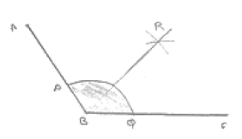Steps of construction:

1. Draw angle ABC of 120°

2. With center B and any radius, draw an arc which intersects AB at P and BC at Q

3. With center P and Q and radius more than 1/2 PQ, draw two arcs, with intersect each other at R.

4. Join BR

∴ ∠ABB = ∠RBC = 60°

​Question: 3

Using your protractor, thaw an angle of measure 108°. With this angle as given, draw an angle of 54°.

Solution: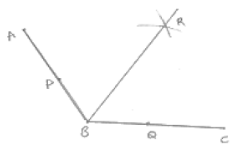Steps of construction:

1. Draw an angle ABC of 108°

2. With center B and any radius, draw an arc which intersects AB at P and BC at Q

3. With center P and Q and radius more than 2 PQ, draw two arcs, which intersect each

other at R.

4. Join BR

∴ ∠RBC = 54°

​Question: 4

Using protractor, draw a right angle. Bisect it to get an angle of measure 45°.

Solution: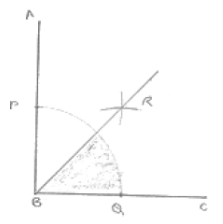Steps of construction:

1. Draw an angle ABC of 90°

2. With center B and any radius, draw an arc which intersects AB at P and BC at Q

3. With center P and Q and radius more than 1/2 PQ, draw two arcs, which intersect each 2 other at R.

4. Join RB

∴ ∠RBC = 45°

​Question: 5

Draw a linear pair of angles. Bisect each of the two angles. Verify that the two bisecting rays are perpendicular to each other.

Solution:Steps of construction:

1. Draw two angle DCA and DCB forming Linear pair

2. With center C and any radius, draw an arc which intersects AC at P, CD at Q and CB at R.

3. With center P and Q and any radius draw two arcs which interest each other at S

4. Join SC

5. With center Q and R any radius draw two arcs, which intersect each other at T.

6. Join TC

∠SCT = 90° [By using protractor]

​Question: 6

Draw a pair of vertically opposite angles. Bisect each of the two angles. Verify that the bisecting rays are in the same line.

Solution: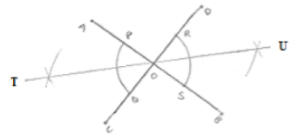Steps of construction:

1. Draw a pair of vertically opposite angle AOC and DOB

2. With center 0 and any radius drawn two arcs which intersect OA at P, Q - OB at S and OD at R.

3. With center P and Q and radius more than 1/2 PQ, draw two arcs which intersect each other at U.

4. Join to

5. With center R and S radius more than 1/2 RS, draw two arcs which intersect each other at U.

6. Join OU.

∴ TOU is a straight line

​Question: 7

Using ruler and compasses only, draw a right angle.

Solution: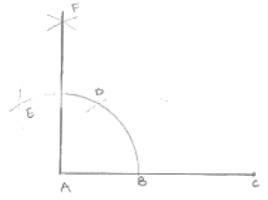Steps of construction:

1. Draw a line segment AB

2. With center A and any radius draw arc which intersect AB at C.

3. With center C and same radius draw an arc which intersects AB at C.

4. With center D and same radius draw arc which intersect arc in (2) at E.

5. With centers E and C and any radius, draw two arcs which intersect each other at F.

6. Join FA

∠FAB = 90°

​Question: 8

Using ruler and compasses only, draw an angle of measure 135°.

Solution: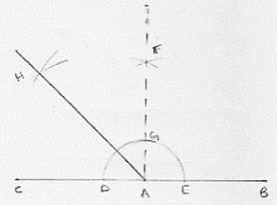Steps of construction:

1. Draw a line segment AB and produce BA to point C.

2. With center A and any radius draw arc which intersect AC at D and AB at E.

3. With center D and E and radius more than 1/2 DE, draw two arcs which intersect each other at F.

4. Join FA which intersect the arc in (2) at G.

5. With centers G and D and radius more than 1/2 GD, draw two arcs which intersect each other at H.

6. Join HA

∴ ∠HAB = 135°

​Question: 9

Using a protractor, draw an angle of measure 72°. With this angle as given, draw angles of measure 36° and 54°.

Solution:

Steps of construction: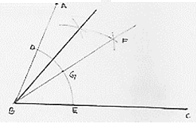1. Draw an angle ABC of 72° with the help of protractor.

2. With center B and any radius, draw an arc which intersect AB at D and BC at E.

3. With center D and E and radius more than 1/2 DE, draw two arcs which intersect each other at F.

4. Join FB which intersect the arc in (2) at G.

5. With centers D and G and radius more than 1/2 DE, draw two arcs which intersect each other at F.

6. With centers D and G and radius more than n 1/2 DG draw two arcs which intersect each other at H

7. Join HB

∴ ∠HBC = 54°

∠FBC = 36°

​Question: 10

Construct the following angles at the initial point of a given ray and justify the construction:

(i) 45°

(ii) 90°

Solution: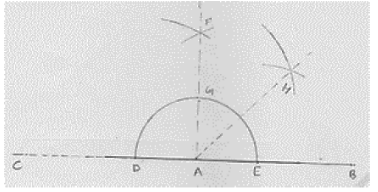(i) Steps of construction:

1. Draw a line segment AB and produce BA to point C.

2. With center A and any radius drawn an arc which intersect AC at D and AB at E.

3. With center D and E and radius more than 1/2 DE, draw arcs cutting each other at F.

4. Join FA which intersect arc in (2) at G.

5. With centers G and E and radius more than 1/2 GE, draw arcs cutting each other at H.

6. Join HA

∴ ∠HAB = 45°

(ii) Steps of construction: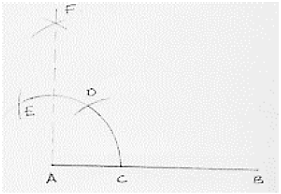1. Draw a line segment AB.

2. With center A and any radius draw in arc which intersect AB at C.

3. With center C and same radius thaw an arc which intersects previous arc at D.

4. With centers D same radius draw an arc which intersects are in (2) at E.

5. With centers E and D same radius more than 1/2 ED draw an arc cutting each other at F.

6. Join FA

∴ ∠FAB = 90°
```### Course Features

• 728 Video Lectures
• Revision Notes
• Previous Year Papers
• Mind Map
• Study Planner
• NCERT Solutions
• Discussion Forum
• Test paper with Video Solution